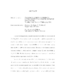## Transmon qubits coupled to superconducting lumped element resonators2015
##### Authors
I discuss the design, fabrication and measurement at millikelvin-temperatures of Al/AlO$_x$/Al Josephson junction-based transmon qubits coupled to superconducting thin-film lumped element microwave resonators made of aluminum on sapphire. The resonators had a center frequency of around $6\,$GHz, and a total quality factor ranging from 15,000 to 70,000 for the various devices. The area of the transmon junctions was about $150\, \mathrm{nm} \times 150\, \mathrm{nm}$ and with Josephson energy $E_J$ such that $10\,\text{GHz} \leq E_J / h \leq 30\,$GHz. The charging energy of the transmons arising mostly from the large interdigital shunt capacitance, was $E_c / h \approx 300\,$MHz. I present microwave spectroscopy of the devices in the strongly dispersive regime of circuit quantum electrodynamics. In this limit the ac Stark shift due to a single photon in the resonator is greater than the linewidth of the qubit transition. When the resonator is driven coherently using a coupler tone, the transmon spectrum reveals individual photon number'' peaks, each corresponding to a single additional photon in the resonator. Using a weighted average of the peak heights in the qubit spectrum, I calculated the average number of photons $\bar{n}$ in the resonator. I also observed a nonlinear variation of $\bar{n}$ with the applied power of the coupler tone $P_{rf}$. I studied this nonlinearity using numerical simulations and found good qualitative agreement with data. In the absence of a coherent drive on the resonator, a thermal population of $5.474\,$GHz photons in the resonator, at an effective temperature of $120\,$mK resulted in a weak $n=1$ thermal photon peak in the qubit spectrum. In the presence of independent coupler and probe tones, the $n=1$ thermal photon peak revealed an Autler-Townes splitting. The observed effect was explained accurately using the four lowest levels of the dispersively dressed Jaynes-Cummings transmon-resonator system, and numerical simulations of the steady-state master equation for the coupled system. I also present time-domain measurements on transmons coupled to lumped-element resonators. From $T_1$ and Rabi oscillation measurements, I found that my early transmon devices (called design LEv5) had lifetimes ($T_1 \sim 1\,\mu$s) limited by strong coupling to the $50\,\Omega$ transmission line. This coupling was characterized by the the rate of change of the Rabi oscillation frequency with the change in the drive voltage ($\mathrm{d}f_{Rabi}\, / \mathrm{d}V$) -- also termed the Rabi coupling to the drive. I studied the design of the transmon-resonator system using circuit analysis and microwave simulations with the aim being to reduce the Rabi coupling to the drive. By increasing the resonance frequency of the resonator $\omega_r/2\pi$ from 5.4$\,$GHz to 7.2$\,$GHz, lowering the coupling of the resonator to the transmission line and thereby increasing the external quality factor $Q_e$ from 20,000 to 70,000, and reducing the transmon-resonator coupling $g/2\pi$ from 70$\,$MHz to 40$\,$MHz, I reduced the Rabi coupling to the drive by an order of magnitude ($\sim$ factor of 20). The $T_1 \sim 4\,\mu$s of devices in the new design (LEv6) was longer than that of the early devices, but still much shorter than the lifetimes predicted from Rabi coupling, suggesting the presence of alternative sources of noise causing qubit relaxation. Microwave simulations and circuit analysis in the presence of a dielectric loss tangent $\tan \delta \simeq 5\times10^{-6}$ agree reasonably well with the measured $T_1$ values, suggesting that surface dielectric loss may be causing relaxation of transmons in the new designs.